Courses

# Test: Previous Year Questions - Binomial Numbers (Competition Level 2)

## 14 Questions MCQ Test Mathematics For JEE | Test: Previous Year Questions - Binomial Numbers (Competition Level 2)

Description
This mock test of Test: Previous Year Questions - Binomial Numbers (Competition Level 2) for JEE helps you for every JEE entrance exam. This contains 14 Multiple Choice Questions for JEE Test: Previous Year Questions - Binomial Numbers (Competition Level 2) (mcq) to study with solutions a complete question bank. The solved questions answers in this Test: Previous Year Questions - Binomial Numbers (Competition Level 2) quiz give you a good mix of easy questions and tough questions. JEE students definitely take this Test: Previous Year Questions - Binomial Numbers (Competition Level 2) exercise for a better result in the exam. You can find other Test: Previous Year Questions - Binomial Numbers (Competition Level 2) extra questions, long questions & short questions for JEE on EduRev as well by searching above.
QUESTION: 1

### If in the expansion of (1 + x)m (1 – x)n, the co-efficients of x and x2 are 3 and – 6 respectively, then m is [JEE 99,2 ]

Solution:

(1+x)m (1−x)n = (1 + mx + m(m−1)x2/2!)(1 − nx + n(n−1)x2/2! )
= 1 + (m−n)x + [(n2 − n)/2 − mn + (m2 − m)/2] x2
Given m − n = 3 or n = m − 3
Hence (n2 − n)/2 − mn + (m2 − m)/2 = 6
⇒ [(m−3)(m−4)]/2 − m(m−3) + (m2 − m)/2 = −6
⇒ m2 − 7m + 12 − 2m2 + 6m + m2 − m + 12 = 0
⇒ − 2m + 24 = 0
⇒ m = 12.

QUESTION: 2

Solution:
QUESTION: 3

### Find the largest co-efficient in the expansion of (1 + x)n, given that the sum of co-efficients of the terms in its expansion is 4096.     [REE 2000 (Mains)]

Solution:

We know that, the coefficients in a binomial expansion is obtained by replacing each variable by unit in the given expression.
Therefore, sum of the coefficients in (a+b)^n
= 4096=(1+1)n
⇒ 4096=(2)n
⇒ (2)12=(2)n
⇒ n=12
Here n is even, so the greatest coefficient is nCn/2  i.e., 12C6

QUESTION: 4

In the binomial expansion of (a - b)n, n ³ 5, the sum of the 5th and 6th terms is zero. Then equals.

Solution:

It is given that T6​+T5​=0.
Hence nC4an−4b4−nC5an−5b5=0
nC4​an−4b4= nC5an−5b5
nC4​a = nC5b
n!b/(n−4)!4! = n!b/(n−5)!5!
= a/(n−4) = b/5​
Therefore a/b=(n−4)/5

QUESTION: 5

Find the coefficient of x49 in the polynomial [REE 2001 (Mains), 3]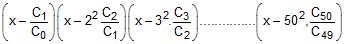where Cr = 50Cr

Solution:
QUESTION: 6

The sum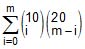, (where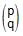= O if P < q) is maximum when m is [JEE 2002 (Scr.), 3]

Solution: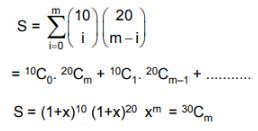QUESTION: 7

(a) Coefficient of t24 in the expansion of (1 + t2)12 (1 + t12) (1 + t24) is [JEE 2003 (Scr.), 3]

(b) Prove that :[JEE 2003 (Mains),2]

Solution:

(1+x)12 (1+x12) (1+x24)
= [C0 + C1x2 + C2x4 + C3x6 + C4x8 +.......C12x24) (1 + x12 + x24 + x36)
= x24(12C0 + 12C6 + 12C12)
= 1 + 12C6 + 1
= 12C6  + 2

QUESTION: 8

n_1Cr = (k2 – 3). nCr + 1if k Î       [JEE 2004 (Scr.)]

Solution:

Formula,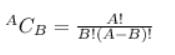(n - 1)!/{r! × (n - 1 - r)!} = (k² - 3) × n!/(r + 1)!(n - r - 1)!
or, (n - 1)!/r! = (k² - 3) × n!/(r + 1)!
or, (n - 1)!/r! = (k² - 3) × n(n - 1)!/(r + 1)r!
or, 1/1 = (k² - 3) × n/(r + 1)
or, (r + 1)/n = (k² - 3)
we know, r and n are integers so, (r + 1)/n  (0, 1]
So, 0 < (r + 1)/n ≤ 1
or, 0 < k² - 3 ≤ 1
or, 3 < k² ≤ 4
or, √3 < k ≤ 2 , -2 ≤ k < -√3
Hence, k  (√3, 2]

QUESTION: 9

n-1Cr = (k2 - 3). nCr + 1 [JEE 2004 (Scr.)]

Solution:

(n - 1)!/{r! × (n - 1 - r)!} = (k² - 3) × n!/(r + 1)!(n - r - 1)!
or, (n - 1)!/r! = (k² - 3) × n!/(r + 1)!
or, (n - 1)!/r! = (k² - 3) × n(n - 1)!/(r + 1)r!
or, 1/1 = (k² - 3) × n/(r + 1)
or, (r + 1)/n = (k² - 3)
we know, r and n are integers so, (r + 1)/n  (0, 1]
so, 0 < (r + 1)/n ≤ 1
or, 0 < k² - 3 ≤ 1
or, 3 < k² ≤ 4
or, √3 < k ≤ 2 , -2 ≤ k < -√3
hence, k  (√3, 2]

QUESTION: 10

The value of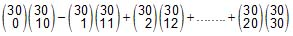is, where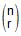= nCr        [JEE 2005 (Scr.)]

Solution: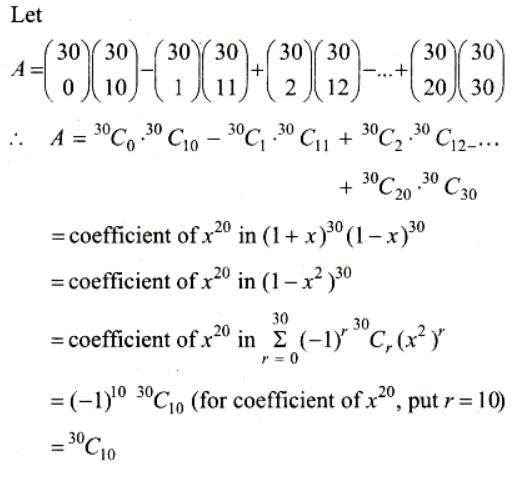QUESTION: 11

The number of seven digit integers, with sum of the digits equal to 10 and formed by using the digits 1, 2 and 3 only, is               [JEE 2009]

Solution:
QUESTION: 12

For r = 0, 1, ...., 10 let Ar, Br, Cr denote, respectively, the coefficient of xr in the expansions of (1 + x)10, (1 + x)20 and (1 + x)30. Then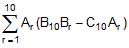is equal to       [JEE 2010]

Solution:
QUESTION: 13

Let an denote the number of all n-digit positive integers formed by the digits 0, 1 or both such that no consecutive digits in them are 0. Let bn = the number of such n-digit integers ending with digit 1 and cn = the number of such n-digit integers ending with digit 0.         [JEE 2012]

Which of the following is correct ?

Solution:
QUESTION: 14

Let an denote the number of all n-digit positive integers formed by the digits 0, 1 or both such that no consecutive digits in them are 0. Let bn = the number of such n-digit integers ending with digit 1 and cn = the number of such n-digit integers ending with digit 0.                 [JEE 2012]

The value of b6 is

Solution: